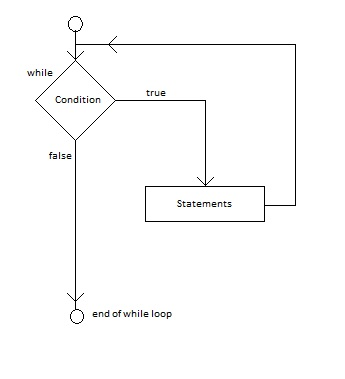# While Loop in C# programming

While Loop is a loop in programming languages like C# that repeats a block of statements until a given condition is true. The condition comes after while and it can be any expression that return boolean value. The expression inside the while loop is executed only if the condition is satisfied. The condition can be changed inside the loop. The loop exits when the condition is false.

### Syntax for while loop:

```while (condition)
{
statements;
}```

### Flow chart for while loop:### Example 1: C# example for while loop

C# Program to display multiplication table for given number.

```using System;
namespace loop
{
class Program
{
static void Main(string[] args)
{
Console.Write("Enter a number: ");
int i = 1; //initialization
while (i <= 10) //condition
{
Console.WriteLine(a + " * " + i + " = " + i*a);
i++; //increment
}
}
}
}```

The above program gets a number from user. Then, the variable “i” to be used in while loop is initialized. The condition is checked, and if it is true it enters the loop and prints the multiplication. The variable is then incremented and the loop repeated till i is less than or equal to 10. This way, the program display multiplication of any given number.

Output:

```Enter a number: 6
6 * 1 = 6
6 * 2 = 12
6 * 3 = 18
6 * 4 = 24
6 * 5 = 30
6 * 6 = 36
6 * 7 = 42
6 * 8 = 48
6 * 9 = 54
6 * 10 = 60
```

### Additional Information of while Loop

If the condition in the loop is not changing, the loop will run forever and it is known as infinite loop. So, we must put increment or decrement inside the loop. Or we can use break operator when we need to exit the loop.